# Introductory Examples¶

## Overview¶

We’re now ready to start learning the Julia language itself.

### Level¶

Our approach is aimed at those who already have at least some knowledge of programming — perhaps experience with Python, MATLAB, Fortran, C or similar.

In particular, we assume you have some familiarity with fundamental programming concepts such as

• variables
• arrays or vectors
• loops
• conditionals (if/else)

### Approach¶

In this lecture we will write and then pick apart small Julia programs.

At this stage the objective is to introduce you to basic syntax and data structures.

Deeper concepts—how things work—will be covered in later lectures.

Since we are looking for simplicity the examples are a little contrived

In this lecture, we will often start with a direct MATLAB/FORTRAN approach which often is poor coding style in Julia, but then move towards more elegant code which is tightly connected to the mathematics.

### Set Up¶

We assume that you’ve worked your way through our getting started lecture already.

In particular, the easiest way to install and precompile all the Julia packages used in QuantEcon notes is to type ] add InstantiateFromURL and then work in a Jupyter notebook, as described here.

### Other References¶

The definitive reference is Julia’s own documentation.

The manual is thoughtfully written but is also quite dense (and somewhat evangelical).

The presentation in this and our remaining lectures is more of a tutorial style based around examples.

## Example: Plotting a White Noise Process¶

To begin, let’s suppose that we want to simulate and plot the white noise process $\epsilon_0, \epsilon_1, \ldots, \epsilon_T$, where each draw $\epsilon_t$ is independent standard normal.

### Introduction to Packages¶

The first step is to activate a project environment, which is encapsulated by Project.toml and Manifest.toml files.

There are three ways to install packages and versions (where the first two methods are discouraged, since they may lead to package versions out-of-sync with the notes)

1. add the packages directly into your global installation (e.g. Pkg.add("MyPackage") or ] add MyPackage)
2. download an Project.toml and Manifest.toml file in the same directory as the notebook (i.e. from the @__DIR__ argument), and then call using Pkg; Pkg.activate(@__DIR__);
3. use the InstantiateFromURL package
In :
using InstantiateFromURL
# optionally add arguments to force installation: instantiate = true, precompile = true
github_project("QuantEcon/quantecon-notebooks-julia", version = "0.8.0")


If you have never run this code on a particular computer, it is likely to take a long time as it downloads, installs, and compiles all dependent packages.

This code will download and install project files from the lecture repo.

We will discuss it more in Tools and Editors, but these files provide a listing of packages and versions used by the code.

This ensures that an environment for running code is reproducible, so that anyone can replicate the precise set of package and versions used in construction.

The careful selection of package versions is crucial for reproducibility, as otherwise your code can be broken by changes to packages out of your control.

After the installation and activation, using provides a way to say that a particular code or notebook will use the package.

In :
using LinearAlgebra, Statistics


### Using Functions from a Package¶

Some functions are built into the base Julia, such as randn, which returns a single draw from a normal distibution with mean 0 and variance 1 if given no parameters.

In :
randn()

Out:
-1.1100477539931022

Other functions require importing all of the names from an external library

In :
using Plots
gr(fmt=:png); # setting for easier display in jupyter notebooks

n = 100
ϵ = randn(n)
plot(1:n, ϵ)

Out: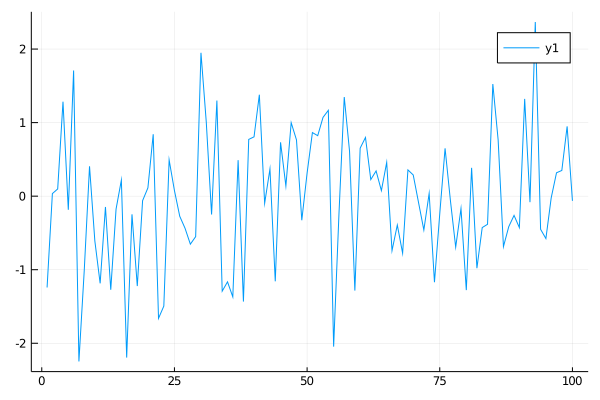Let’s break this down and see how it works.

The effect of the statement using Plots is to make all the names exported by the Plots module available.

Because we used Pkg.activate previously, it will use whatever version of Plots.jl that was specified in the Project.toml and Manifest.toml files.

The other packages LinearAlgebra and Statistics are base Julia libraries, but require an explicit using.

The arguments to plot are the numbers 1,2, ..., n for the x-axis, a vector ϵ for the y-axis, and (optional) settings.

The function randn(n) returns a column vector n random draws from a normal distribution with mean 0 and variance 1.

### Arrays¶

As a language intended for mathematical and scientific computing, Julia has strong support for using unicode characters.

In the above case, the ϵ and many other symbols can be typed in most Julia editor by providing the LaTeX and <TAB>, i.e. \epsilon<TAB>.

The return type is one of the most fundamental Julia data types: an array

In :
typeof(ϵ)

Out:
Array{Float64,1}
In :
ϵ[1:5]

Out:
5-element Array{Float64,1}:
-1.2418433428846225
0.034815280479051795
0.097936162596475
1.2843849964476541
-0.18536959442283946

The information from typeof() tells us that ϵ is an array of 64 bit floating point values, of dimension 1.

In Julia, one-dimensional arrays are interpreted as column vectors for purposes of linear algebra.

The ϵ[1:5] returns an array of the first 5 elements of ϵ.

Notice from the above that

• array indices start at 1 (like MATLAB and Fortran, but unlike Python and C)
• array elements are referenced using square brackets (unlike MATLAB and Fortran)

To get help and examples in Jupyter or other julia editor, use the ? before a function name or syntax.

?typeof

search: typeof typejoin TypeError

Get the concrete type of x.

Examples

julia> a = 1//2;

julia> typeof(a)
Rational{Int64}

julia> M = [1 2; 3.5 4];

julia> typeof(M)
Array{Float64,2}


### For Loops¶

Although there’s no need in terms of what we wanted to achieve with our program, for the sake of learning syntax let’s rewrite our program to use a for loop for generating the data.

Note

In Julia v0.7 and up, the rules for variables accessed in for and while loops can be sensitive to how they are used (and variables can sometimes require a global as part of the declaration). We strongly advise you to avoid top level (i.e. in the REPL or outside of functions) for and while loops outside of Jupyter notebooks. This issue does not apply when used within functions.

Starting with the most direct version, and pretending we are in a world where randn can only return a single value

In :
# poor style
n = 100
ϵ = zeros(n)
for i in 1:n
ϵ[i] = randn()
end


Here we first declared ϵ to be a vector of n numbers, initialized by the floating point 0.0.

The for loop then populates this array by successive calls to randn().

Like all code blocks in Julia, the end of the for loop code block (which is just one line here) is indicated by the keyword end.

The word in from the for loop can be replaced by either ∈ or =.

The index variable is looped over for all integers from 1:n – but this does not actually create a vector of those indices.

Instead, it creates an iterator that is looped over – in this case the range of integers from 1 to n.

While this example successfully fills in ϵ with the correct values, it is very indirect as the connection between the index i and the ϵ vector is unclear.

To fix this, use eachindex

In :
# better style
n = 100
ϵ = zeros(n)
for i in eachindex(ϵ)
ϵ[i] = randn()
end


Here, eachindex(ϵ) returns an iterator of indices which can be used to access ϵ.

While iterators are memory efficient because the elements are generated on the fly rather than stored in memory, the main benefit is (1) it can lead to code which is clearer and less prone to typos; and (2) it allows the compiler flexibility to creatively generate fast code.

In Julia you can also loop directly over arrays themselves, like so

In :
ϵ_sum = 0.0 # careful to use 0.0 here, instead of 0
m = 5
for ϵ_val in ϵ[1:m]
ϵ_sum = ϵ_sum + ϵ_val
end
ϵ_mean = ϵ_sum / m

Out:
0.6462327258740999

where ϵ[1:m] returns the elements of the vector at indices 1 to m.

Of course, in Julia there are built in functions to perform this calculation which we can compare against

In :
ϵ_mean ≈ mean(ϵ[1:m])
ϵ_mean ≈ sum(ϵ[1:m]) / m

Out:
true

In these examples, note the use of ≈ to test equality, rather than ==, which is appropriate for integers and other types.

Approximately equal, typed with \approx<TAB>, is the appropriate way to compare any floating point numbers due to the standard issues of floating point math.

### User-Defined Functions¶

For the sake of the exercise, let’s go back to the for loop but restructure our program so that generation of random variables takes place within a user-defined function.

To make things more interesting, instead of directly plotting the draws from the distribution, let’s plot the squares of these draws

In :
# poor style
function generatedata(n)
ϵ = zeros(n)
for i in eachindex(ϵ)
ϵ[i] = (randn())^2 # squaring the result
end
return ϵ
end

data = generatedata(10)
plot(data)

Out: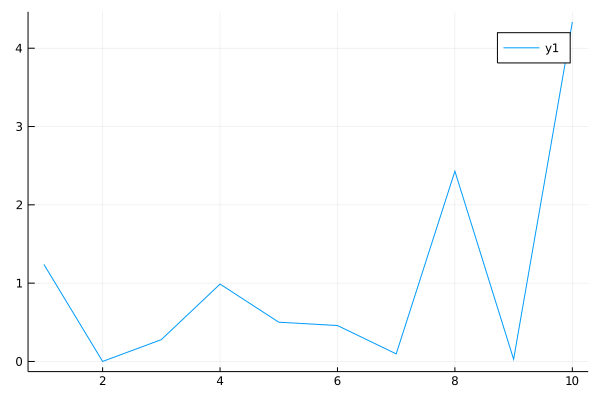Here

• function is a Julia keyword that indicates the start of a function definition
• generatedata is an arbitrary name for the function
• return is a keyword indicating the return value, as is often unnecessary

Let us make this example slightly better by “remembering” that randn can return a vectors.

In :
# still poor style
function generatedata(n)
ϵ = randn(n) # use built in function

for i in eachindex(ϵ)
ϵ[i] = ϵ[i]^2 # squaring the result
end

return ϵ
end
data = generatedata(5)

Out:
5-element Array{Float64,1}:
5.899343334685265e-5
2.7013292493691687
0.16482905026631123
0.4654169462547691
0.11479031913761378

While better, the looping over the i index to square the results is difficult to read.

Instead of looping, we can broadcast the ^2 square function over a vector using a ..

To be clear, unlike Python, R, and MATLAB (to a lesser extent), the reason to drop the for is not for performance reasons, but rather because of code clarity.

Loops of this sort are at least as efficient as vectorized approach in compiled languages like Julia, so use a for loop if you think it makes the code more clear.

In :
# better style
function generatedata(n)
ϵ = randn(n) # use built in function
return ϵ.^2
end
data = generatedata(5)

Out:
5-element Array{Float64,1}:
4.222226913497673
0.00021621170414375565
6.1477590127192
1.5211032220967127
0.04802586215468419

We can even drop the function if we define it on a single line.

In :
# good style
generatedata(n) = randn(n).^2
data = generatedata(5)

Out:
5-element Array{Float64,1}:
1.5975060667542837
0.00010504247374677952
0.0922752271698986
0.2872874054833071
0.14902112700768044

Finally, we can broadcast any function, where squaring is only a special case.

In :
# good style
f(x) = x^2 # simple square function
generatedata(n) = f.(randn(n)) # uses broadcast for some function f
data = generatedata(5)

Out:
5-element Array{Float64,1}:
10.758319037101021
2.837540169374459
0.45372028461056974
0.360555253618547
0.012889014767242376

As a final – abstract – approach, we can make the generatedata function able to generically apply to a function.

In :
generatedata(n, gen) = gen.(randn(n)) # uses broadcast for some function gen

f(x) = x^2 # simple square function
data = generatedata(5, f) # applies f

Out:
5-element Array{Float64,1}:
0.3252704877135321
0.0015107695272444906
0.7243858609405561
7.290455211280642
0.078307306608254

Whether this example is better or worse than the previous version depends on how it is used.

High degrees of abstraction and generality, e.g. passing in a function f in this case, can make code either clearer or more confusing, but Julia enables you to use these techniques with no performance overhead.

For this particular case, the clearest and most general solution is probably the simplest.

In :
# direct solution with broadcasting, and small user-defined function
n = 100
f(x) = x^2

x = randn(n)
plot(f.(x), label="x^2")
plot!(x, label="x") # layer on the same plot

Out: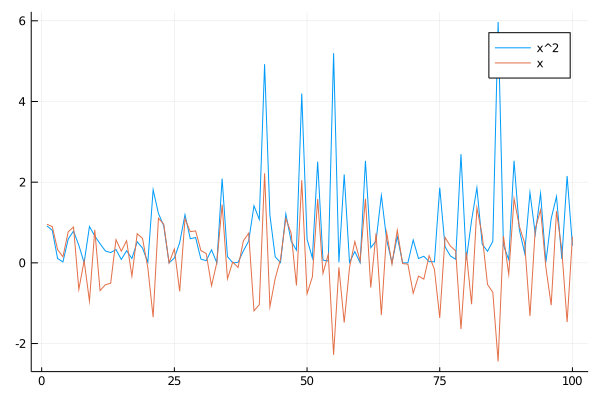While broadcasting above superficially looks like vectorizing functions in MATLAB, or Python ufuncs, it is much richer and built on core foundations of the language.

The other additional function plot! adds a graph to the existing plot.

This follows a general convention in Julia, where a function that modifies the arguments or a global state has a ! at the end of its name.

#### A Slightly More Useful Function¶

Let’s make a slightly more useful function.

This function will be passed in a choice of probability distribution and respond by plotting a histogram of observations.

In doing so we’ll make use of the Distributions package, which we assume was instantiated above with the project.

Here’s the code

In :
using Distributions

function plothistogram(distribution, n)
ϵ = rand(distribution, n)  # n draws from distribution
histogram(ϵ)
end

lp = Laplace()
plothistogram(lp, 500)

Out: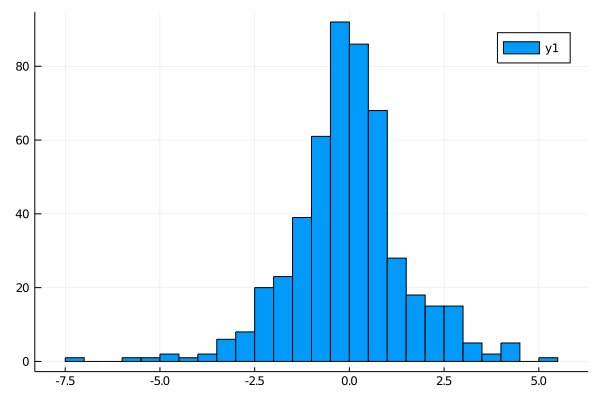Let’s have a casual discussion of how all this works while leaving technical details for later in the lectures.

First, lp = Laplace() creates an instance of a data type defined in the Distributions module that represents the Laplace distribution.

The name lp is bound to this value.

When we make the function call plothistogram(lp, 500) the code in the body of the function plothistogram is run with

• the name distribution bound to the same value as lp
• the name n bound to the integer 500

#### A Mystery¶

Now consider the function call rand(distribution, n).

This looks like something of a mystery.

The function rand() is defined in the base library such that rand(n) returns n uniform random variables on $[0, 1)$.

In :
rand(3)

Out:
3-element Array{Float64,1}:
0.10127440130185938
0.8674278247892253
0.2651255434123343

On the other hand, distribution points to a data type representing the Laplace distribution that has been defined in a third party package.

So how can it be that rand() is able to take this kind of value as an argument and return the output that we want?

The answer in a nutshell is multiple dispatch, which Julia uses to implement generic programming.

This refers to the idea that functions in Julia can have different behavior depending on the particular arguments that they’re passed.

Hence in Julia we can take an existing function and give it a new behavior by defining how it acts on a new type of value.

The compiler knows which function definition to apply to in a given setting by looking at the types of the values the function is called on.

In Julia these alternative versions of a function are called methods.

## Example: Variations on Fixed Points¶

Take a mapping $f : X \to X$ for some set $X$.

If there exists an $x^* \in X$ such that $f(x^*) = x^*$, then $x^*$: is called a “fixed point” of $f$.

For our second example, we will start with a simple example of determining fixed points of a function.

The goal is to start with code in a MATLAB style, and move towards a more Julian style with high mathematical clarity.

### Fixed Point Maps¶

Consider the simple equation, where the scalars $p,\beta$ are given, and $v$ is the scalar we wish to solve for

$$v = p + \beta v$$

Of course, in this simple example, with parameter restrictions this can be solved as $v = p/(1 - \beta)$.

Rearrange the equation in terms of a map $f(x) : \mathbb R \to \mathbb R$

$$v = f(v) \tag{1}$$

where

$$f(v) := p + \beta v$$

Therefore, a fixed point $v^*$ of $f(\cdot)$ is a solution to the above problem.

### While Loops¶

One approach to finding a fixed point of (1) is to start with an initial value, and iterate the map

$$v^{n+1} = f(v^n) \tag{2}$$

For this exact f function, we can see the convergence to $v = p/(1-\beta)$ when $|\beta| < 1$ by iterating backwards and taking $n\to\infty$

$$v^{n+1} = p + \beta v^n = p + \beta p + \beta^2 v^{n-1} = p \sum_{i=0}^{n-1} \beta^i + \beta^n v_0$$

To implement the iteration in (2), we start by solving this problem with a while loop.

The syntax for the while loop contains no surprises, and looks nearly identical to a MATLAB implementation.

In :
# poor style
p = 1.0 # note 1.0 rather than 1
β = 0.9
maxiter = 1000
tolerance = 1.0E-7
v_iv = 0.8 # initial condition

# setup the algorithm
v_old = v_iv
normdiff = Inf
iter = 1
while normdiff > tolerance && iter <= maxiter
v_new = p + β * v_old # the f(v) map
normdiff = norm(v_new - v_old)

# replace and continue
v_old = v_new
iter = iter + 1
end
println("Fixed point = $v_old, and |f(x) - x| =$normdiff in $iter iterations")  Fixed point = 9.999999173706609, and |f(x) - x| = 9.181037796679448e-8 in 155 iterations  The while loop, like the for loop should only be used directly in Jupyter or the inside of a function. Here, we have used the norm function (from the LinearAlgebra base library) to compare the values. The other new function is the println with the string interpolation, which splices the value of an expression or variable prefixed by \$ into a string.

An alternative approach is to use a for loop, and check for convergence in each iteration.

In :
# setup the algorithm
v_old = v_iv
normdiff = Inf
iter = 1
for i in 1:maxiter
v_new = p + β * v_old # the f(v) map
normdiff = norm(v_new - v_old)
if normdiff < tolerance # check convergence
iter = i
break # converged, exit loop
end
# replace and continue
v_old = v_new
end
println("Fixed point = $v_old, and |f(x) - x| =$normdiff in $iter iterations")  Fixed point = 9.999999081896231, and |f(x) - x| = 9.181037796679448e-8 in 154 iterations  The new feature there is break , which leaves a for or while loop. ### Using a Function¶ The first problem with this setup is that it depends on being sequentially run – which can be easily remedied with a function. In : # better, but still poor style function v_fp(β, ρ, v_iv, tolerance, maxiter) # setup the algorithm v_old = v_iv normdiff = Inf iter = 1 while normdiff > tolerance && iter <= maxiter v_new = p + β * v_old # the f(v) map normdiff = norm(v_new - v_old) # replace and continue v_old = v_new iter = iter + 1 end return (v_old, normdiff, iter) # returns a tuple end # some values p = 1.0 # note 1.0 rather than 1 β = 0.9 maxiter = 1000 tolerance = 1.0E-7 v_initial = 0.8 # initial condition v_star, normdiff, iter = v_fp(β, p, v_initial, tolerance, maxiter) println("Fixed point =$v_star, and |f(x) - x| = $normdiff in$iter iterations")

Fixed point = 9.999999173706609, and |f(x) - x| = 9.181037796679448e-8 in 155 iterations


While better, there could still be improvements.

### Passing a Function¶

The chief issue is that the algorithm (finding a fixed point) is reusable and generic, while the function we calculate p + β * v is specific to our problem.

A key feature of languages like Julia, is the ability to efficiently handle functions passed to other functions.

In :
# better style
function fixedpointmap(f, iv, tolerance, maxiter)
# setup the algorithm
x_old = iv
normdiff = Inf
iter = 1
while normdiff > tolerance && iter <= maxiter
x_new = f(x_old) # use the passed in map
normdiff = norm(x_new - x_old)
x_old = x_new
iter = iter + 1
end
return (x_old, normdiff, iter)
end

# define a map and parameters
p = 1.0
β = 0.9
f(v) = p + β * v # note that p and β are used in the function!

maxiter = 1000
tolerance = 1.0E-7
v_initial = 0.8 # initial condition

v_star, normdiff, iter = fixedpointmap(f, v_initial, tolerance, maxiter)
println("Fixed point = $v_star, and |f(x) - x| =$normdiff in $iter iterations")  Fixed point = 9.999999173706609, and |f(x) - x| = 9.181037796679448e-8 in 155 iterations  Much closer, but there are still hidden bugs if the user orders the settings or returns types wrong. ### Named Arguments and Return Values¶ To enable this, Julia has two features: named function parameters, and named tuples In : # good style function fixedpointmap(f; iv, tolerance=1E-7, maxiter=1000) # setup the algorithm x_old = iv normdiff = Inf iter = 1 while normdiff > tolerance && iter <= maxiter x_new = f(x_old) # use the passed in map normdiff = norm(x_new - x_old) x_old = x_new iter = iter + 1 end return (value = x_old, normdiff=normdiff, iter=iter) # A named tuple end # define a map and parameters p = 1.0 β = 0.9 f(v) = p + β * v # note that p and β are used in the function! sol = fixedpointmap(f, iv=0.8, tolerance=1.0E-8) # don't need to pass println("Fixed point =$(sol.value), and |f(x) - x| = $(sol.normdiff) in$(sol.iter)"*
" iterations")

Fixed point = 9.999999918629035, and |f(x) - x| = 9.041219328764782e-9 in 177 iterations


In this example, all function parameters after the ; in the list, must be called by name.

Furthermore, a default value may be enabled – so the named parameter iv is required while tolerance and maxiter have default values.

The return type of the function also has named fields, value, normdiff, and iter – all accessed intuitively using ..

To show the flexibilty of this code, we can use it to find a fixed point of the non-linear logistic equation, $x = f(x)$ where $f(x) := r x (1-x)$.

In :
r = 2.0
f(x) = r * x * (1 - x)

sol = fixedpointmap(f, iv=0.8)
println("Fixed point = $(sol.value), and |f(x) - x| =$(sol.normdiff) in $(sol.iter) iterations")  Fixed point = 0.4999999999999968, and |f(x) - x| = 3.979330237546819e-8 in 7 iterations  ### Using a Package¶ But best of all is to avoid writing code altogether. In : # best style using NLsolve p = 1.0 β = 0.9 f(v) = p .+ β * v # broadcast the + sol = fixedpoint(f, [0.8]) println("Fixed point =$(sol.zero), and |f(x) - x| = $(norm(f(sol.zero) - sol.zero)) in " * "$(sol.iterations) iterations")

Fixed point = [9.999999999999973], and |f(x) - x| = 3.552713678800501e-15 in 3 iterations


The fixedpoint function from the NLsolve.jl library implements the simple fixed point iteration scheme above.

Since the NLsolve library only accepts vector based inputs, we needed to make the f(v) function broadcast on the + sign, and pass in the initial condition as a vector of length 1 with [0.8].

While a key benefit of using a package is that the code is clearer, and the implementation is tested, by using an orthogonal library we also enable performance improvements.

In :
# best style
p = 1.0
β = 0.9
iv = [0.8]
sol = fixedpoint(v -> p .+ β * v, iv)
println("Fixed point = $(sol.zero), and |f(x) - x| =$(norm(f(sol.zero) - sol.zero)) in " *
"$(sol.iterations) iterations")  Fixed point = [9.999999999999973], and |f(x) - x| = 3.552713678800501e-15 in 3 iterations  Note that this completes in 3 iterations vs 177 for the naive fixed point iteration algorithm. Since Anderson iteration is doing more calculations in an iteration, whether it is faster or not would depend on the complexity of the f function. But this demonstrates the value of keeping the math separate from the algorithm, since by decoupling the mathematical definition of the fixed point from the implementation in (2), we were able to exploit new algorithms for finding a fixed point. The only other change in this function is the move from directly defining f(v) and using an anonymous function. Similar to anonymous functions in MATLAB, and lambda functions in Python, Julia enables the creation of small functions without any names. The code v -> p .+ β * v defines a function of a dummy argument, v with the same body as our f(x). ### Composing Packages¶ A key benefit of using Julia is that you can compose various packages, types, and techniques, without making changes to your underlying source. As an example, consider if we want to solve the model with a higher-precision, as floating points cannot be distinguished beyond the machine epsilon for that type (recall that computers approximate real numbers to the nearest binary of a given precision; the machine epsilon is the smallest nonzero magnitude). In Julia, this number can be calculated as In : eps()  Out: 2.220446049250313e-16 For many cases, this is sufficient precision – but consider that in iterative algorithms applied millions of times, those small differences can add up. The only change we will need to our model in order to use a different floating point type is to call the function with an arbitrary precision floating point, BigFloat, for the initial value. In : # use arbitrary precision floating points p = 1.0 β = 0.9 iv = [BigFloat(0.8)] # higher precision # otherwise identical sol = fixedpoint(v -> p .+ β * v, iv) println("Fixed point =$(sol.zero), and |f(x) - x| = $(norm(f(sol.zero) - sol.zero)) in " * "$(sol.iterations) iterations")

Fixed point = BigFloat[10.00000000000000222044604925031357388532909931412848377287867809936811555686155], and |f(x) - x| = 0.0 in 3 iterations


Here, the literal BigFloat(0.8) takes the number 0.8 and changes it to an arbitrary precision number.

The result is that the residual is now exactly 0.0 since it is able to use arbitrary precision in the calculations, and the solution has a finite-precision solution with those parameters.

### Multivariate Fixed Point Maps¶

The above example can be extended to multivariate maps without any modifications to the fixed point iteration code.

Using our own, homegrown iteration and simply passing in a bivariate map:

In :
p = [1.0, 2.0]
β = 0.9
iv = [0.8, 2.0]
f(v) = p .+ β * v # note that p and β are used in the function!

sol = fixedpointmap(f, iv = iv, tolerance = 1.0E-8)
println("Fixed point = $(sol.value), and |f(x) - x| =$(sol.normdiff) in $(sol.iter)"* "iterations")  Fixed point = [9.999999961080519, 19.999999923853192], and |f(x) - x| = 9.501826248250528e-9 in 184iterations  This also works without any modifications with the fixedpoint library function. In : using NLsolve p = [1.0, 2.0, 0.1] β = 0.9 iv =[0.8, 2.0, 51.0] f(v) = p .+ β * v sol = fixedpoint(v -> p .+ β * v, iv) println("Fixed point =$(sol.zero), and |f(x) - x| = $(norm(f(sol.zero) - sol.zero)) in " * "$(sol.iterations) iterations")

Fixed point = [10.0, 20.000000000000004, 0.9999999999999929], and |f(x) - x| = 6.661338147750939e-16 in 3 iterations


Finally, to demonstrate the importance of composing different libraries, use a StaticArrays.jl type, which provides an efficient implementation for small arrays and matrices.

In :
using NLsolve, StaticArrays
p = @SVector [1.0, 2.0, 0.1]
β = 0.9
iv = [0.8, 2.0, 51.0]
f(v) = p .+ β * v

sol = fixedpoint(v -> p .+ β * v, iv)
println("Fixed point = $(sol.zero), and |f(x) - x| =$(norm(f(sol.zero) - sol.zero)) in " *
"$(sol.iterations) iterations")  Fixed point = [10.0, 20.000000000000004, 0.9999999999999929], and |f(x) - x| = 6.661338147750939e-16 in 3 iterations  The @SVector in front of the [1.0, 2.0, 0.1] is a macro for turning a vector literal into a static vector. All macros in Julia are prefixed by @ in the name, and manipulate the code prior to compilation. We will see a variety of macros, and discuss the “metaprogramming” behind them in a later lecture. ## Exercises¶ ### Exercise 1¶ Recall that$ n! $is read as “$ n $factorial” and defined as$ n! = n \times (n - 1) \times \cdots \times 2 \times 1 $. In Julia you can compute this value with factorial(n). Write your own version of this function, called factorial2, using a for loop. ### Exercise 2¶ The binomial random variable$ Y \sim Bin(n, p) $represents • number of successes in$ n $binary trials • each trial succeeds with probability$ p $Using only rand() from the set of Julia’s built-in random number generators (not the Distributions package), write a function binomial_rv such that binomial_rv(n, p) generates one draw of$ Y $. Hint: If$ U $is uniform on$ (0, 1) $and$ p \in (0,1) $, then the expression U < p evaluates to true with probability$ p $. ### Exercise 3¶ Compute an approximation to$ \pi $using Monte Carlo. For random number generation use only rand(). Your hints are as follows: • If$ U $is a bivariate uniform random variable on the unit square$ (0, 1)^2 $, then the probability that$ U $lies in a subset$ B $of$ (0,1)^2 $is equal to the area of$ B $. • If$ U_1,\ldots,U_n $are iid copies of$ U $, then, as$ n $gets larger, the fraction that falls in$ B $converges to the probability of landing in$ B $. • For a circle, area = π *$ radius^2 $. ### Exercise 4¶ Write a program that prints one realization of the following random device: • Flip an unbiased coin 10 times. • If 3 consecutive heads occur one or more times within this sequence, pay one dollar. • If not, pay nothing. Once again use only rand() as your random number generator. ### Exercise 5¶ Simulate and plot the correlated time series $$x_{t+1} = \alpha \, x_t + \epsilon_{t+1} \quad \text{where} \quad x_0 = 0 \quad \text{and} \quad t = 0,\ldots,n$$ The sequence of shocks$ \{\epsilon_t\} $is assumed to be iid and standard normal. Set$ n = 200 $and$ \alpha = 0.9 $. ### Exercise 6¶ Plot three simulated time series, one for each of the cases$ \alpha = 0 $,$ \alpha = 0.8 $and$ \alpha = 0.98 $. (The figure will illustrate how time series with the same one-step-ahead conditional volatilities, as these three processes have, can have very different unconditional volatilities) ### Exercise 7¶ This exercise is more challenging. Take a random walk, starting from$ x_0 = 1 $$$x_{t+1} = \, \alpha \, x_t + \sigma\, \epsilon_{t+1} \quad \text{where} \quad x_0 = 1 \quad \text{and} \quad t = 0,\ldots,t_{\max}$$ • Furthermore, assume that the$ x_{t_{\max}} = 0 $(i.e. at$ t_{\max} $, the value drops to zero, regardless of its current state). • The sequence of shocks$ \{\epsilon_t\} $is assumed to be iid and standard normal. • For a given path$ \{x_t\} $define a first-passage time as$ T_a = \min\{t\, |\, x_t \leq a\} $, where by the assumption of the process$ T_a \leq t_{\max} $. Start with$ \sigma = 0.2, \alpha = 1.0 $1. calculate the first-passage time,$ T_0 $, for 100 simulated random walks – to a$ t_{\max} = 200 $and plot a histogram 2. plot the sample mean of$ T_0 $from the simulation for$ \alpha \in \{0.8, 1.0, 1.2\} $### Exercise 8(a)¶ This exercise is more challenging. The root of a univariate function$ f(\cdot) $is an$ x $such that$ f(x) = 0 $. One solution method to find local roots of smooth functions is called Newton’s method. Starting with an$ x_0 $guess, a function$ f(\cdot) $and the first-derivative$ f'(\cdot) $, the algorithm is to repeat $$x^{n+1} = x^n - \frac{f(x^n)}{f'(x^n)}$$ until$ | x^{n+1} - x^n| $is below a tolerance 1. Use a variation of the fixedpointmap code to implement Newton’s method, where the function would accept arguments f, f_prime, x_0, tolerance, maxiter. 2. Test it with$ f(x) = (x-1)^3 $and another function of your choice where you can analytically find the derivative. ### Exercise 8(b)¶ For those impatient to use more advanced features of Julia, implement a version of Exercise 8(a) where f_prime is calculated with auto-differentiation. In : using ForwardDiff # operator to get the derivative of this function using AD D(f) = x -> ForwardDiff.derivative(f, x) # example usage: create a function and get the derivative f(x) = x^2 f_prime = D(f) f(0.1), f_prime(0.1)  Out: (0.010000000000000002, 0.2) 1. Using the D(f) operator definition above, implement a version of Newton’s method that does not require the user to provide an analytical derivative. 2. Test the sorts of f functions which can be automatically integrated by ForwardDff.jl. ## Solutions¶ ### Exercise 1¶ In : function factorial2(n) k = 1 for i in 1:n k *= i # or k = k * i end return k end factorial2(4)  Out: 24 In : factorial2(4) == factorial(4) # built-in function  Out: true ### Exercise 2¶ In : function binomial_rv(n, p) count = 0 U = rand(n) for i in 1:n if U[i] < p count += 1 # or count = count + 1 end end return count end for j in 1:25 b = binomial_rv(10, 0.5) print("$b, ")
end

4, 3, 4, 5, 5, 6, 3, 4, 5, 6, 6, 4, 6, 2, 3, 5, 3, 6, 6, 9, 1, 7, 4, 3, 5,

### Exercise 3¶

Consider a circle with diameter 1 embedded in a unit square.

Let $A$ be its area and let $r = 1/2$ be its radius.

If we know $\pi$ then we can compute $A$ via $A = \pi r^2$.

But the point here is to compute $\pi$, which we can do by $\pi = A / r^2$.

Summary: If we can estimate the area of the unit circle, then dividing by $r^2 = (1/2)^2 = 1/4$ gives an estimate of $\pi$.

We estimate the area by sampling bivariate uniforms and looking at the fraction that fall into the unit circle.

In :
n = 1000000
count = 0
for i in 1:n
u, v = rand(2)
d = sqrt((u - 0.5)^2 + (v - 0.5)^2)  # distance from middle of square
if d < 0.5
count += 1
end
end

area_estimate = count / n

print(area_estimate * 4)  # dividing by radius**2

3.142144

### Exercise 4¶

In :
payoff = 0
count = 0

print("Count = ")

for i in 1:10
U = rand()
if U < 0.5
count += 1
else
count = 0
end
print(count)
if count == 3
payoff = 1
end
end
println("\npayoff = $payoff")  Count = 1200012300 payoff = 1  We can simplify this somewhat using the ternary operator. Here are some examples In : a = 1 < 2 ? "foo" : "bar"  Out: "foo" In : a = 1 > 2 ? "foo" : "bar"  Out: "bar" Using this construction: In : payoff = 0.0 count = 0.0 print("Count = ") for i in 1:10 U = rand() count = U < 0.5 ? count + 1 : 0 print(count) if count == 3 payoff = 1 end end println("\npayoff =$payoff")

Count = 0012012345
payoff = 1


### Exercise 5¶

Here’s one solution

In :
using Plots
gr(fmt=:png); # setting for easier display in jupyter notebooks
α = 0.9
n = 200
x = zeros(n + 1)

for t in 1:n
x[t+1] = α * x[t] + randn()
end
plot(x)

Out: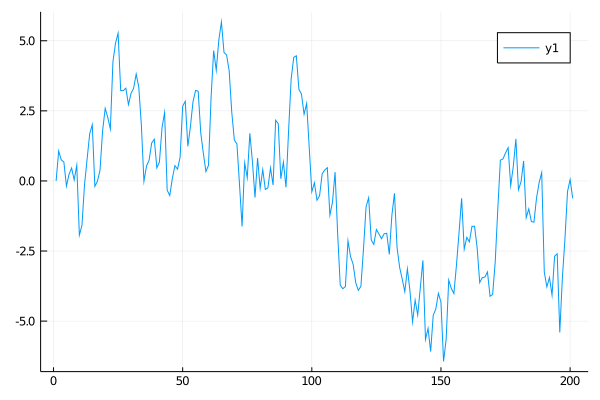### Exercise 6¶

In :
αs = [0.0, 0.8, 0.98]
n = 200
p = plot() # naming a plot to add to

for α in αs
x = zeros(n + 1)
x = 0.0
for t in 1:n
x[t+1] = α * x[t] + randn()
end
plot!(p, x, label = "alpha = $α") # add to plot p end p # display plot  Out: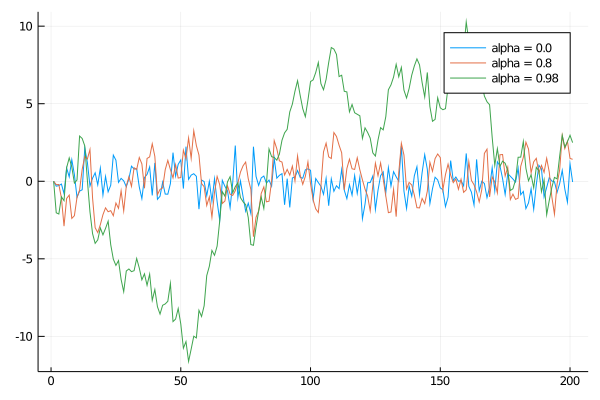### Exercise 7: Hint¶ As a hint, notice the following pattern for finding the number of draws of a uniform random number until it is below a given threshold In : function drawsuntilthreshold(threshold; maxdraws=100) for i in 1:maxdraws val = rand() if val < threshold # checks threshold return i # leaves function, returning draw number end end return Inf # if here, reached maxdraws end draws = drawsuntilthreshold(0.2, maxdraws=100)  Out: 10 Additionally, it is sometimes convenient to add to just push numbers onto an array without indexing it directly In : vals = zeros(0) # empty vector for i in 1:100 val = rand() if val < 0.5 push!(vals, val) end end println("There were$(length(vals)) below 0.5")

There were 43 below 0.5